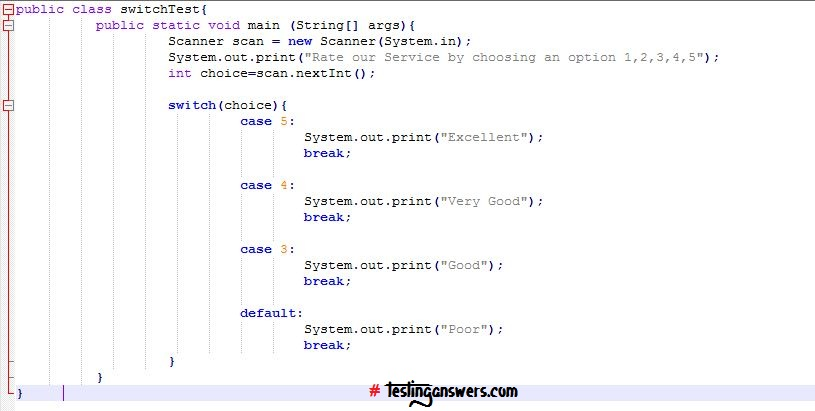# 09.1 Control FlowJava, like any other programming language, supports both conditional and loop statements to control code flow.

9.1 | Conditional Statements

In Java, we have four conditional statements:

1. if(condition=true){}: Execute a set of code when the specified condition is true.

2. if(condition=true){}else{}: Execute first block of code when the specified condition is true else executes the second block of code.

3. if(condition1=true){}elseif{condition2=true}{}else{}: Execute the first block of code for which the condition is true. If no condition is found true and else block exists, then this block of code is executed. If no condition is found true and else block does not exist, then none of the if block code is executed.

4. Switch(choice)…case: Executes a set of code for which the choice condition is true. switch…case is used when the number of available alternatives and options are many and at a time only one holds true. Though the same can be implemented using if condition too but that makes the code cumbersome and less readable.

9.1.1 | if…Statement

Consider a scenario where code logic to be implemented is as follows:
• If the specified number is less than 5, then print “number<5”.
• Elseif the specified number is >=5 and <10, then print “number is between 5 and 10”.
• Else, print “number is >=10”.9.1.2 | switch…Statement

Consider a scenario where the code logic to be implemented is as follows:
• If grade = 5, then print “Excellent”.
• If grade = 4, then print “Very Good”.
• If grade = 3, then print “Good”.
• Else, print “Poor”.